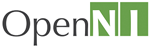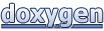# OpenNI 1.5.4: XnArray< T > Class Template Reference

#### OpenNIOpenNI 1.5.4
XnArray< T > Class Template Reference

`#include <XnArray.h>`

List of all members.

## Public Types

enum  { BASE_SIZE = 8 }
typedef T * Iterator
typedef const T * ConstIterator

## Public Member Functions

XnArray (XnUInt32 nBaseSize=BASE_SIZE)
XnArray (const XnArray &other)
virtual ~XnArray ()
XnArrayoperator= (const XnArray &other)
XnStatus CopyFrom (const XnArray &other)
XnStatus SetData (const T *pData, XnUInt32 nSize)
const T * GetData () const
T * GetData ()
XnStatus Reserve (XnUInt32 nReservedSize)
XnBool IsEmpty () const
XnUInt32 GetSize () const
XnStatus SetSize (XnUInt32 nSize)
XnStatus SetSize (XnUInt32 nSize, const T &fillVal)
XnStatus SetMinSize (XnUInt32 nSize)
XnStatus SetMinSize (XnUInt32 nSize, const T &fillVal)
XnUInt32 GetAllocatedSize () const
XnStatus Set (XnUInt32 nIndex, const T &val)
XnStatus Set (XnUInt32 nIndex, const T &val, const T &fillVal)
XnStatus AddLast (const T *aValues, XnUInt32 nCount)
void Clear ()
T & operator[] (XnUInt32 nIndex)
const T & operator[] (XnUInt32 nIndex) const
Iterator begin ()
ConstIterator begin () const
Iterator end ()
ConstIterator end () const

## Member Typedef Documentation

template<typename T>
 typedef const T* XnArray< T >::ConstIterator

A constant iterator object that allows you to iterate over members in the array as read-only values.

template<typename T>
 typedef T* XnArray< T >::Iterator

An iterator object that allows you to iterate over members in the array.

## Member Enumeration Documentation

template<typename T>
 anonymous enum
Enumerator:
 BASE_SIZE

## Constructor & Destructor Documentation

template<typename T>
 XnArray< T >::XnArray ( XnUInt32 nBaseSize = `BASE_SIZE` ) ` [inline]`

Default constructor. Initializes the array to BASE_SIZE.

template<typename T>
 XnArray< T >::XnArray ( const XnArray< T > & other ) ` [inline]`

Copy constructor. Initializes this array from another array of the same type.

template<typename T>
 virtual XnArray< T >::~XnArray ( ) ` [inline, virtual]`

Destructor. Deallocates data in this array.

## Member Function Documentation

template<typename T>
 XnStatus XnArray< T >::AddLast ( const T & val ) ` [inline]`

Adds one element to the end of this array.

template<typename T>
 XnStatus XnArray< T >::AddLast ( const T * aValues, XnUInt32 nCount ) ` [inline]`

Adds a range of values to the end of this array.

template<typename T>
 Iterator XnArray< T >::begin ( ) ` [inline]`
Returns:
a modifiable iterator pointing to the beginning of this array.
template<typename T>
 ConstIterator XnArray< T >::begin ( ) const` [inline]`
Returns:
a const iterator pointing to the beginning of this array.
template<typename T>
 void XnArray< T >::Clear ( ) ` [inline]`

Resets the data of this array and sets its size to 0.

template<typename T>
 XnStatus XnArray< T >::CopyFrom ( const XnArray< T > & other ) ` [inline]`

Copies data from another array of the same type.

template<typename T>
 Iterator XnArray< T >::end ( ) ` [inline]`
Returns:
a modifiable itertor pointing to the end of this array.
template<typename T>
 ConstIterator XnArray< T >::end ( ) const` [inline]`
Returns:
a const itertor pointing to the end of this array.
template<typename T>
 XnUInt32 XnArray< T >::GetAllocatedSize ( ) const` [inline]`
Returns:
The allocated size of this array. This is the maximum number of elements that the array can hold without re-allocating and copying data.
template<typename T>
 const T* XnArray< T >::GetData ( ) const` [inline]`
Returns:
the raw data of this array as a read-only buffer.
template<typename T>
 T* XnArray< T >::GetData ( ) ` [inline]`
Returns:
the raw data of this array as a modifiable buffer.
template<typename T>
 XnUInt32 XnArray< T >::GetSize ( ) const` [inline]`
Returns:
The number of elements in this array.
template<typename T>
 XnBool XnArray< T >::IsEmpty ( ) const` [inline]`
Returns:
TRUE if this array is empty, FALSE otherwise.
template<typename T>
 XnArray& XnArray< T >::operator= ( const XnArray< T > & other ) ` [inline]`

Assignment operator. Copies data from another array of the same type.

template<typename T>
 T& XnArray< T >::operator[] ( XnUInt32 nIndex ) ` [inline]`

Element access operator. Returns a modifiable reference to the element at specified index.

template<typename T>
 const T& XnArray< T >::operator[] ( XnUInt32 nIndex ) const` [inline]`

Element access operator. Returns a const reference to the element at specified index.

template<typename T>
 XnStatus XnArray< T >::Reserve ( XnUInt32 nReservedSize ) ` [inline]`

Reserves space in this array for the specified number of elements. This saves you re-allocations and data copies if you know the size in advance.

template<typename T>
 XnStatus XnArray< T >::Set ( XnUInt32 nIndex, const T & val ) ` [inline]`

Sets value at specified index, grows array if needed. Note that when dealing with primitive types and adding beyond the current size of the array, any added values that fill the gap are uninitialized

template<typename T>
 XnStatus XnArray< T >::Set ( XnUInt32 nIndex, const T & val, const T & fillVal ) ` [inline]`

Sets value at specified index and grows array if needed, filling any resulting blank elements with fillVal.

template<typename T>
 XnStatus XnArray< T >::SetData ( const T * pData, XnUInt32 nSize ) ` [inline]`

Copies data from a raw buffer of the same type pointed to by pData, and sets size to nSize.

template<typename T>
 XnStatus XnArray< T >::SetMinSize ( XnUInt32 nSize ) ` [inline]`

Makes sure the array has at least nSize elements. If nSize is less than the current size, there is no effect. Note that for primitive types, any added elements are uninitialized.

template<typename T>
 XnStatus XnArray< T >::SetMinSize ( XnUInt32 nSize, const T & fillVal ) ` [inline]`

Makes sure the array has at least nSize elements. If nSize is less than the current size, there is no effect. Any added elements are initialized by the value specified by fillVal.

template<typename T>
 XnStatus XnArray< T >::SetSize ( XnUInt32 nSize ) ` [inline]`

Sets the size of this array to the specified number of elements. Note that for primitive types, the data is uninitialized.

template<typename T>
 XnStatus XnArray< T >::SetSize ( XnUInt32 nSize, const T & fillVal ) ` [inline]`

Sets the size of this array to the specified number of elements, initializing all elements with the value specified by fillVal.

The documentation for this class was generated from the following file:
Generated on Wed May 16 2012 10:16:06 for OpenNI 1.5.4 by1.7.5.1